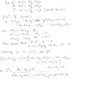# Vector question-

fahd

Given two vectors A and B and a scalar 'd', it is known that:
A.C=d and A X C = B
where C is a vector of unknown direction and magnitude.Find an expression for C in terms of A,B,d and the magnitude of vector A.

I tried using langranges identity but am getting a value of c's magnitude and not C as a vector..Like I am getting sumthing like
c^2=(B^2+d^2)/B^2 which i know is kinda wrong as the answer iv got is a magnitude and not a vector..
What do i do?Please help!Last edited:

Staff Emeritus
Gold Member
I'd go with component representations of each vector ($\vec{A}=A_x\hat{i}+A_y\hat{j}+A_z\hat{k}$, etc.) Now if you write out $\vec{A}\times\vec{C}=\vec{B}$ you'll get a 3x3 system of equations for the components of $\vec{C}$. It will look tempting to solve the system, but you won't be able to (the coefficient matrix is singular). But you could use 2 of those equations, and for the third equation use $\vec{A}\cdot\vec{C}=d$. Then you should be able to solve for the components of $\vec{C}$. Once you have those, you're done.

Last edited:
fahd
Tom Mattson said:
I'd go with component representations of each vector ($\vec{A}=A_x\hat{i}+A_y\hat{j}+A_z\hat{k}$, etc.) Now if you write out $\vec{A}\times\vec{C}=\vec{B}$ you'll get a 3x3 system of equations for the components of $\vec{C}$. It will look tempting to solve the system, but you won't be able to (the coefficient matrix is singular). But you could use 2 of those equations, and for the third equation use $\vec{A}\cdot\vec{C}=d$. Then you should be able to solve for the components of $\vec{C}$. Once you have those, you're done.

hi..i tried doing the question and have got an unusual answer..shown on the included attachment..The question said that the answer shud be in terms of A,B,d and magnitude of A.However mine isn't coming as shown..Plz help!Thanks!

#### Attachments

•scan0002.jpg
25.4 KB · Views: 297
Last edited:
Staff Emeritus
Gold Member
I think you're going to have to flex your algebra muscles a little more. I really don't feel like solving the whole thing, but for $C_x$ I get:

$$C_x=\frac{da_x-a_yb_z+a_zb_y}{a_x^2+a_y^2+a_z^2}$$
$$C_x=\frac{da_x-(\vec{A}\times\vec{B})_x}{|\vec{A}|^2}$$.

If the other components go by that pattern, and if I haven't made any dumb mistakes, then it should follow that:

$$\vec{C}=\frac{d\vec{A}-\vec{A}\times\vec{B}}{|\vec{A}|^2}$$.

Try to work it out, OK?

balakrishnan_v
A.C=D
AxC=B
So we have
$$(AXC)XA=|A|^2 C-(C.A)A=BXA$$

So
$$C=\frac{BXA+dA}{|A|^2}$$

fahd
heey..i tried working it now and got it...u knw what..the only thing was that everything seemed so abstract that it was confusing me like nething..i mean unknown components etc..
nehow..thanks again..
i finally got it!